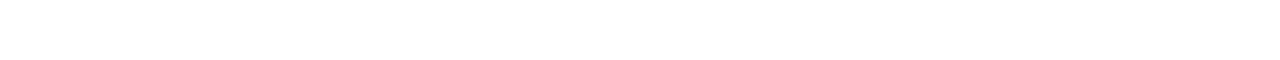# 15.1.4 Gravitational Field Strength

### Deriving Gravitational Field Strength (g)

• The gravitational field strength at a point describes how strong or weak a gravitational field is at that point
• The gravitational field strength due to a point mass can be derived from combining the equations for Newton’s law of gravitation and gravitational field strength
• For calculations involving gravitational forces, a spherical mass can be treated as a point mass at the centre of the sphere
• Newton’s law of gravitation states that the attractive force F between two masses M and m with separation r is equal to:• Cancelling mass m, the equation becomes:• Where:
• g = gravitational field strength (N kg-1)
• G = Newton’s Gravitational Constant
• M = mass of the body producing the gravitational field (kg)
• r = distance from the mass where you are calculating the field strength (m)

### Calculating g

• Gravitational field strength, g, is a vector quantity
• The direction of g is always towards the centre of the body creating the gravitational field
• This is the same direction as the gravitational field lines
• On the Earth’s surface, g has a constant value of 9.81 N kg-1
• However outside the Earth’s surface, g is not constant
• g decreases as r increases by a factor of 1/r2
• This is an inverse square law relationship with distance
• When g is plotted against the distance from the centre of a planet, r has two parts:
• When r < R, the radius of the planet, g is directly proportional to r
• When r > R, g is inversely proportional to r2 (this is an ‘L’ shaped curve and shows that g decreases rapidly with increasing distance r)
• Sometimes, g is referred to as the ‘acceleration due to gravity’ with units of m s-2
• Any object that falls freely in a uniform gravitational field on Earth has an acceleration of 9.81 m s-2

#### Worked example: Gravitational field strengthStep 1:            Write down the known quantitiesgM = gravitational field strength on the Moon, ρM = mean density of the Moon

gE = gravitational field strength on the Earth, ρE = mean density of the Earth

Step 2:            The volumes of the Earth and Moon are equal to the volume of a sphereStep 3:            Write the density equation and rearrange for mass MM = ρV### Author: Katie

Katie has always been passionate about the sciences, and completed a degree in Astrophysics at Sheffield University. She decided that she wanted to inspire other young people, so moved to Bristol to complete a PGCE in Secondary Science. She particularly loves creating fun and absorbing materials to help students achieve their exam potential.
CloseClose